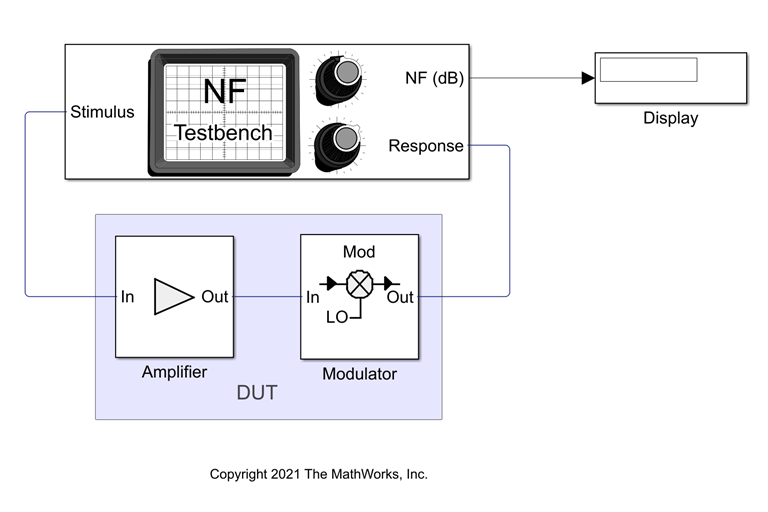# Measure Noise Figure of Device Under Test

Use the Noise Figure Testbench block to measure the noise figure of a device under test (DUT).

Connect the blocks as shown in the model.Set the parameters for DUT and the testbench.

Amplifier block:

• Available power gain`10` dB

• Noise type`Noise figure`

• Noise figure (dB)`4` dB

Mixer block:

• Available power gain`5` dB

• Local oscillator frequency`2.0` GHz

• Add Image Reject filter`on`

• Noise figure (dB)`8` dB

• Filter type`Highpass`

• Implementation`Constant per carrier`

• Passband edge frequency`2.05` GHz

Noise Figure Testbench block:

• Input frequency (Hz)`2.1e9`

• Output frequency (Hz)`0.1e9`

Run the model. You will see that the display shows a OIP3 value of `4.817` dB. This value can be verified analytically using the noise figure (NF) equation provided in .

NF_DUT = 10*log10(F_tot) = 10*log10(F_1 + (F_2 – 1)/ A_1)

= 10*log10((10^(4/10)) + (10^(8/10) – 1)/10^(10/10)) = 4.8328 dB

where,

Noise factor of amplifier, F_1 = 10^((4)/10)

Noise factor of mixer, F_2 = 10^((8)/10)

Gain of amplifier, A_1 = 10^(10/10)Razavi, B.. “RF Microelectronics (2nd Edition) (Prentice Hall Communications Engineering and Emerging Technologies Series).” (2011).